Courses

# Young's Modulus, Bulk Modulus and Elastic Moduli Class 11 Notes | EduRev

## JEE : Young's Modulus, Bulk Modulus and Elastic Moduli Class 11 Notes | EduRev

The document Young's Modulus, Bulk Modulus and Elastic Moduli Class 11 Notes | EduRev is a part of the JEE Course Physics For JEE.
All you need of JEE at this link: JEE

Young’s Modulus

For a solid, in the form of a wire or a thin rod, Young’s modulus of elasticity within elastic limit is defined as the ratio of longitudinal stress to longitudinal strain. It is given as: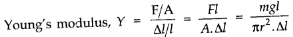It has the unit of longitudinal stress and dimensions of [MI. -1T-2] Its unit is Pascal or N/m2.

Bulk Modulus

Within elastic limit the bulk modulus is defined as the ratio of longitudinal stress and volumetric strain. It is given as: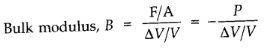– ve indicates that the volume variation and pressure variation always negate each other.

•  Reciprocal of bulk modulus is commonly referred to as the “compressibility”. It is defined as the fractional change in volume per unit change in pressure.
• Shear Modulus or Modulus of Rigidity

It is defined as the ratio of the tangential stress to the shear strain.

Modulus of rigidity is given by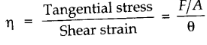•  Poisson’s Ratio

The ratio of change in diameter (ΔD) to the original diameter (D) is called lateral strain. The ratio of change in length (Δl) to the original length (l) is called longitudinal strain. The ratio of lateral strain to the longitudinal strain is called Poisson’s ratio.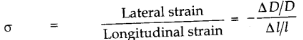For most of the substances, the value of σ lies between 0.2 to 0.4 When a boay is perfectly incompressible, the value of σ is maximum and equals to 0.5.

• Elastic Fatigue

It is the property of an elastic body by virtue of which its behaviour becomes less elastic under the action of repeated alternating deforming forces.

•  Relations between Elastic Moduli

For isotropic materials (i.e., materials having the same properties in all directions), only two of the three elastic constants are independent. For example, Young’s modulus can be expressed in terms of the bulk and shear moduli.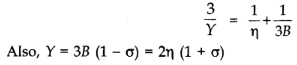•  Breaking Stress

The ultimate tensile strength of a material is the stress required to break a wire or a rod by pulling on it. The breaking stress of the material is the maximum stress which a material can withstand. Beyond this point breakage occurs.

• When a wire of original length L is stretched by a length 1 by the applied I inn of force F at one end, then

Work done to stretch wire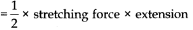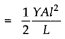• Work done per unit volume of wire is given as: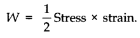According to the formula given by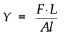Where F is the force needed to stretch the wire of length L and area of cross-section A,  is the increase in the length of the wire.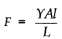The work done by this force in stretching the wire is stored in the wire as potential energy.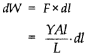Integrating both sides, we get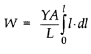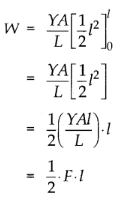Which equal to the elastic potential energy U.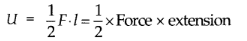Now the potential energy per unit volume is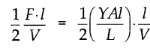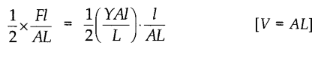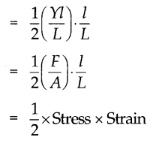Hence, the elastic potential energy of a wire (energy density) is equal to half the product of its stress and strain.

•  IMPORTANT TABLES

TABLE 9.1 Young's modulus, elastic limit and tensile strengths of some materials.

 Substance Young's modulus 109N/m2σy Elastic limit 107N/m2% Tensile strength 107N/m2σu Aluminium 70 IS 20 Copper 120 20 40 Iron {wrought) 190 17 33 Steel 200 30 50 Bone (Tensile) 16 12 (Compressive) 9 12

TABLE 9.2 Shear modulus (G) of some common materials

 Material G(109 Nm-2 or GPa) Aluminium 25 Brass 36 Copper 42 Glass 23 Iron 70 Lead 5.6 Nickel 77 Steel 84 Tungsten 150 Wood 10

TABLE 9.3 Bulk modulus (B) of some common Materials

 Material Solids B(109 Nm-2 or GPa) Aluminium 72 Brass 61 Copper 140 Glass 37 Iron 100 Nickel 260 Steel 160 Liquids Water 2.2 Ethanol 0.9 Carbon disulphide 1.56 Glycerine 4.76 Mercury 25 Gases Air (at 5TP) 1.0 * 10-4

TABLE 9.4 Stress, strain and various elastic modulus

 Type of Stress Stress Strain Change in Elasticmodulus Name of modulus State of Mater shape volume TensileorCompressive Two equal and opposite forces perpendicular to Opposite faces (σ = F/A) Elongation or compression parallel to force direction (ΔL/L) (longitudinal strain) Yes No V = (FxL) /(A » ΔL) Young'smodulus Solid Shearing Two equal and opposite forces parallel to opposite surfaces [forces in each case such that total force and total torque on the body vani-shes (σs * F/A)] Pure shear, θ Yes No C - (F x θ) /A Shearmodulus Solid Hydraulic Forces perpendicular every where to the surface, force per unit area (pressure) same everywhere Volume change (compression or elongation) (ΔV/V) No Yes B = - p/(Δ V) Bulkmodulus Solid,liquid and gas
Offer running on EduRev: Apply code STAYHOME200 to get INR 200 off on our premium plan EduRev Infinity!

## Physics For JEE

187 videos|516 docs|263 tests

,

,

,

,

,

,

,

,

,

,

,

,

,

,

,

,

,

,

,

,

,

,

,

,

;